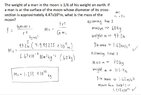# F=g*m1*m2/r^2 related question

Mikasun1108
Homework Statement:
The weight of a man on the moon is 1/6 of his weight on Earth. If a man is at the surface of the moon whose diameter of its cross-section is approximately 4.47x10^6, what is the mass of the moon?
Relevant Equations:
F=gm1m2/r^2
Thanks for the help! :)
Edit: My answer is 1.25 x 10^7 ( I do not think it is correct, I'll try to think some more and update my answer)
Do we need to get the mass of the man? or is this problem actually solvable?
-sun1108

Last edited:

Gold Member
2r=4.47 10^6 meter

Homework Helper
Gold Member
2022 Award
Homework Statement:: The weight of a man on the moon is 1/6 of his weight on Earth. If a man is at the surface of the moon whose diameter of its cross-section is approximately 4.47x10^6, what is the mass of the moon?
Relevant Equations:: F=gm1m2/r^2

Thanks for the help! :)
-sun1108
We need to see your best attempt to solve this yourself.

•Mikasun1108
Mikasun1108
We need to see your best attempt to solve this yourself.
Ook, sorry I didn't put my attempt I wasn't sure about my answer. Ill make sure to write it.

Homework Helper
Gold Member
2022 Award
Edit: My answer is 1.25 x 10^7 ( I do not think it is correct, I'll try to think some more and update my answer)
Looks like an error with the orders of magnitude. Maybe a conversion problem.

Mikasun1108
Looks like an error with the orders of magnitude. Maybe a conversion problem.
Oh right i think i might have place a 667 instead of 6.67. Thanks for the feedback.
I tried doing it again this time I got 1.213 X 10^23 kg
Working: m2= Fr^2/Gm1
Assuming mass is 60kg weight in moon will be 97.2 N
So m2= 97.2N(4.985225 x 10^12m)/6.67 x 10^-11 Nm^2kg^-2(60kg)
m2= 1.213 x 10^23kg

Homework Helper
Gold Member
2022 Award
Oh right i think i might have place a 667 instead of 6.67. Thanks for the feedback.
I tried doing it again this time I got 1.213 X 10^23 kg
Working: m2= Fr^2/Gm1
Assuming mass is 60kg weight in moon will be 97.2 N
So m2= 97.2N(4.985225 x 10^12m)/6.67 x 10^-11 Nm^2kg^-2(60kg)
m2= 1.213 x 10^23kg
When I search online, the mass of the Moon is given as ##7.35 \times 10^{22} kg##.

Homework Helper
Gold Member
2022 Award
PS that said, the diameter of the Moon is given as ##3.5 \times 10^6 m##.

Homework Helper
Gold Member
2022 Award
Using the data you were given, your answer is approximately correct. If I use ##g = 9.81 m/s^2## for Earth, I get ##M = 1.25 \times 10^{23}kg##

Gold Member
PS that said, the diameter of the Moon is given as ##3.5 \times 10^6 m##.
Oh! so re #2, it was not meter but perhaps milli mile ?
[EDIT]
Wrong comment. I confused diameter with radius.

Last edited:
Homework Helper
Gold Member
2022 Award
Oh! so re #2, not meter but mile ?
##m## is metres.

Gold Member
Relevant Equations::[/B] F=gm1m2/r^2
$$GmM_E/6R_E^2=GmM_M/R_M^2$$
$$\frac{\rho_M}{\rho_E} =\frac{1}{6}\frac{ R_E}{R_M}$$
where ##\rho_M,\rho_E## are average density of Moon and the Earth with assumption that both have sphere shape. In observation
$$\frac{\rho_M}{\rho_E} =0.6$$ and $$\frac{ R_E}{R_M} =3.6$$

Last edited:
Mikasun1108
When I search online, the mass of the Moon is given as ##7.35 \times 10^{22} kg##.
So sorry for the very late reply, I haven't been opening my account, thank you so much for your feedback.
I apologize for the very late update: I tried doing the question again later, but then I still arrived at the same answer. Here is my working.

#### Attachments

•IMG-3093.jpg
39.4 KB · Views: 24
Homework Helper
Gold Member
2022 Award
I just put the numbers in a spreadsheet:

 G D R g M 6.67E-11​ 4.47E+06​ 2.24E+06​ 1.635​ 1.22E+23​

The first column is the gravitational constant. The second is the given diameter of the Moon. The third is the radius of the Moon (##R = D/2##). The fourth is the Moon's surface gravity, which I calculated as ##\frac{ 9.81}{6} \ m/s^2##.

The final column, the estimated mass of the Moon, I calculated using the formula for surface gravity ##g = \frac{GM}{R^2}##.

•Mikasun1108
Mikasun1108
I just put the numbers in a spreadsheet:

 G D R g M 6.67E-11​ 4.47E+06​ 2.24E+06​ 1.635​ 1.22E+23​

The first column is the gravitational constant. The second is the given diameter of the Moon. The third is the radius of the Moon (##R = D/2##). The fourth is the Moon's surface gravity, which I calculated as ##\frac{ 9.81}{6} \ m/s^2##.

The final column, the estimated mass of the Moon, I calculated using the formula for surface gravity ##g = \frac{GM}{R^2}##.
Yeyy, so my answer is correct :). Thank you so much for your help and prompt reply, I truly appreciate it :)